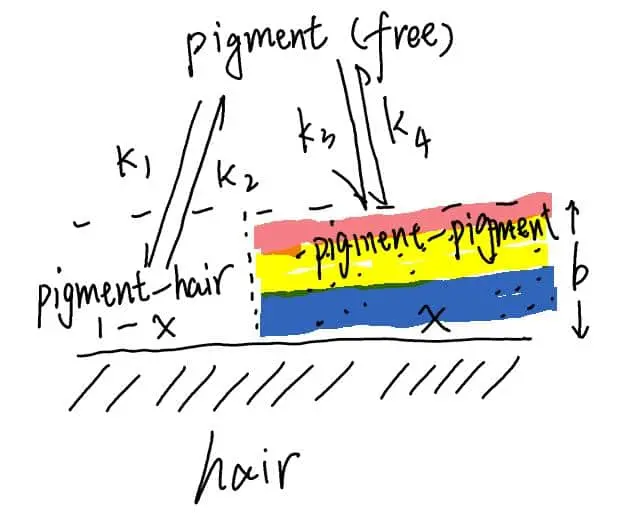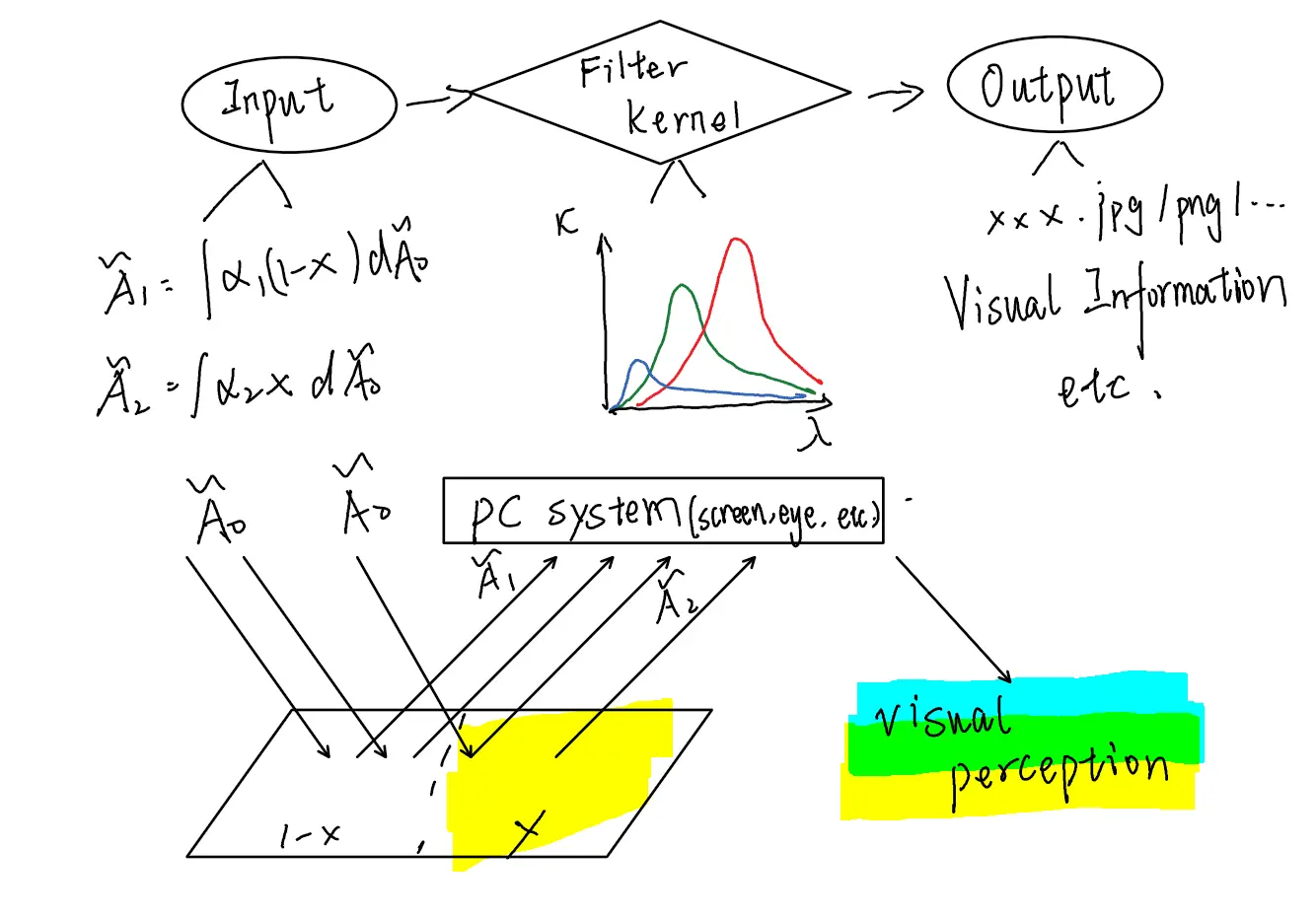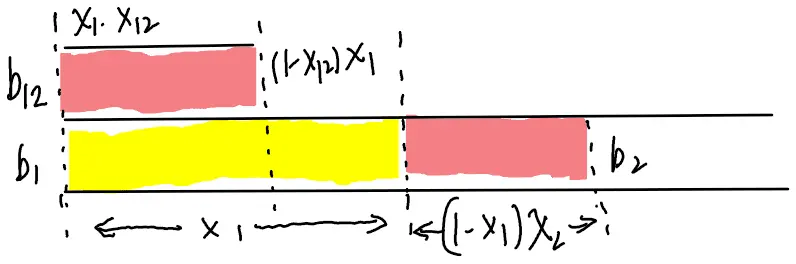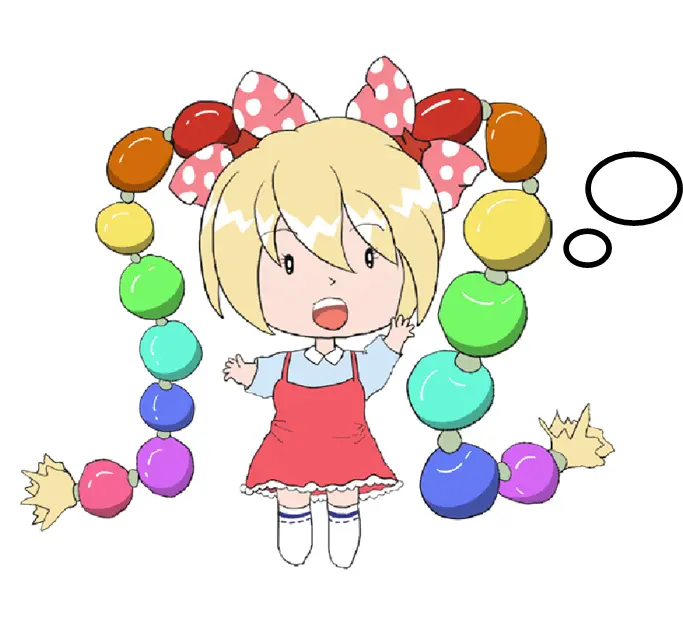# Team:HUST-China/Dye color forecast

Dye color forecast | iGEM HUST-China

# Part 1 Combination of pigment and hair

## 1.1 Hypothesis

• The way hair binds to pigment molecules is based on the interaction of the amino acid naked R group with pigment molecules, including but not limited to hydrogen bonds, Van der Waals Force and so on.
• The interaction between pigment molecules and pigment molecules is much weaker than that between hair and pigment molecules. In other words, we think that the pigment molecules bound to hair are difficult to form a multi-layered structure.
• When dyeing hair, the hair is completely immersed in the dye solution, and the experimental conditions are not enough to make a significant change in the dye solution concentration.

## 1.2 mathematical physics modelFigure 1: mathematical physics model

As shown in the figure, let us suppose that the pigment coverage per unit area is x, and the rate constants for the combination and dissociation of pigment molecules and hair molecules are k1, k2; similarly, the rate constants of the combination and dissociation between the pigment bound to the hair surface and the free pigment molecules are k3 and k4, respectively. t should be noted that the actual meaning of b is controversial, but it must be a measure of the intensity of the surface color, which can be expressed as the thickness of the surface pigment, the molecular number density of the surface pigment and so on. This depends on whether the continuous model or the lattice mosaic model is used to understand the process. We tend to use the lattice mosaic model.

To sum up, there are two ways: $$\ce{Pigment_{(free)} <=>[k_1][k_2] {\ce{Pigment-Hair}}}\tag{1.1}$$ $$\ce{Pigment_{(free)} <=>[k_3][k_4] {\ce{Pigment-Pigment}}}\tag{1.2}$$ The change equation of coverage rate is given by the reaction (1.1) $$\frac{\mathrm{d} x}{\mathrm{d} t} = k_1\Gamma x-k_2n_0(1-x)\tag{1.3}$$ $$\Gamma$$ is the number of collisions between the free pigment molecules and the exposed hair interface per unit time determined by thermodynamics, which is only related to the concentration of pigment molecules, size, solution molecules and temperature, so it is a constant under the experimental conditions. n0 can be understood as the number of pigment molecules embedded in a unit area, which is assumed to be a constant because a single lattice has only two states, mosaic and non-mosaic.

Because the undetermined constants can not be precisely determined, we will explain them as follows:

$$x=1-e^{-pt}\tag{*}$$

p can be considered statistically phenomenologically as the probability per unit time that a pigment molecule binds to a naked hair molecule. The result is physically obvious.

Reaction (1.2) gives the equation of the rate-of-change for intensity measure b :

$$\frac{\mathrm{d} b}{\mathrm{d} t}=k_3\Gamma x-k_4n_0b\tag{1.4}$$

Similarly, $$b = b_0(1-e^{-qt}) + b_1({e^{-pt}-e^{-qt}})\tag{**}$$

# PART 2 Visual Effects of Hair Dyeing

## 2.1 Principles of Colour Vision formationFigure 2: Principles of Colour Vision formation

Take human's vision for example, there are three kinds of vision cells, l, M and S, whose sensitivity to different wavelengths of light is not the same. Therefore, when light enters the human eye, the three kinds of vision cells will convolution according to different sensitivity spectra, and output three different signals to the visual center, the visual center through the value of these three signals, can get the light intensity and its coordinates in the chromaticity space. The color space is a three-dimensional cone space whose scalar value is intensity and whose coordinates correspond to its hue. Whether it is reflection, transmission, absorption, or the visual cells of the "Convolution" operation, are linear transformation in cone space.

Based on this, there is no problem to select RGB value or other index as the base vector in advance, which only means the linear conversion between different base vectors.

## 2.2 Mathematical Representation of Hair Color after Dyeing

Consider a light incident on a hair surface as shown in part 1 and into the human eye. Note the incident light as$\vec A_0$,Considering the share of wavelengths, the incident light may be described as:

$$\vec A_0 = \int_{\Sigma} \vec A_{(\lambda)} d\lambda \tag{2.1}$$

Among them, $$\Sigma$$ is panchromatic space, $$\lambda$$ the wavelength of light, and $$\vec A_{(\lambda)}$$ is the color vision vector of the light corresponding to the monochromatic light.

This is the operation allowed by the color-vision-based cone-space property.

At the same time, we can think that the optical process of light entering the human eye and even the visual cells through the process of reflection and transmission is the superposition of several linear transformations:

$$\vec A = \int_{\Sigma} \alpha_{(\lambda)} \vec A_{(\lambda)} d\lambda \tag{2.2}$$

For a single pigment stain, Part 1 tells you

\begin{aligned} \vec A &= \vec A_1 +\vec A_2\\ &= (1-x)\int_{\Sigma} \alpha_{1(\lambda)} \vec A_{(\lambda)} d\lambda + x\int_{\Sigma} \alpha_{2(\lambda)} \vec A_{(\lambda)} d\lambda\ \end{aligned}

According to (*)

$$x = 1-e^{-pt}$$

we get

$$\vec A = e^{-pt}\vec A_{hair}+(1-e^{-pt})\vec A_{pigment} \tag{2.3}$$ $$And \vec A_{pigment} \ is\ related\ with\ parament\ b\ in\ (**)$$

The complexity of the model is similar to that of Part1, where the effect of the pre-and post-dyeing sequence on the coverage will be taken into account, and the case of both pigments will be mentioned later.

# PART3 Construction of Prediction Model for Dyeing Results

## 3.1 Collection of training sets

We set the appropriate concentration gradient and time gradient, collected the hair dyed under different conditions, and took photos. Finally, the color value is normalized according to the background color.

## 3.2 Monochromatic model structure

Using concentration and time as inputs, set only one layer of hidden layers, three neurons, and activate the function:

$$activation = e^{-x}$$

The reason about choosing such a function is obvious.

## 3.3 Structure of the contamination model

### 3.3.1 Mathematical representation of the mixing modelFigure 3: Mathematical representation of the mixing model

When the first pigment is dyed, there are two systems in the area unit-the pigment-pigment system covering the hair and the naked hair system. If both systems are considered to be similar to those in Part1, the following description is immediately available: $$\begin{matrix} x_1 = 1-e^{-p_1t}\\ x_2 = 1-e^{-p_2t}\\ x_{12} = 1-e^{-p_{12}t}\\ \end{matrix}$$ $$\begin{matrix} b_1 = b_1^0(1-e^{-q_1t}) + b_1^1({e^{-p_1t}-e^{-q_1t}})\\ b_2= b_2^0(1-e^{-q_2t}) + b_2^1({e^{-p_2t}-e^{-q_2t}})\\ b_{12}= b_{12}^0(1-e^{-q_{12}t}) + b_{12}^1(e^{-p_{12}t}-e^{-q_{12}t})\\ \end{matrix}$$

Similarly, give the expression of immediately superior perception:

\begin{aligned} \vec A &= x_1x_{12}\vec A_{pigment12} + x_1(1-x{12}) \vec A_{pigment1} + x_2(1-x_1) \vec A_{pigment2} + (1-x_1)(1-x_2)\vec A_{hair}\\ &=(1-e^{-p_1t})[(1-e^{p_{12}t})\vec A_{pigment12}+e^{-p_{12}t}\vec A_{pigment1}]+e^{-p_2t}[(1-e^{-p_2t})\vec A_{pigment2}+e^{-p_2t}\vec A_{hair}] \end{aligned}

In advance, since the environment of the pigment solution is a known limit value when it is put into use, the parameters such as $$p_1$$, $$p_2$$, $$q_1$$, $$q_2$$ are constant values.

### 3.3.2 Mixing Model

step1 input the pure color hair model in 3.2 according to the type, concentration and dyeing time of pigment, and get two groups of RGB values.

step2 Because of the lattice mosaic model, we think that the system of pigment1-pigment2 contains very little, so we scale the second group RGB values:

$$scaling = (1-e^{-8t_2})$$

Among them, $$t_2$$ (unit: 0.5h) refers to the second dyeing time, exponent 8 is the parameter chosen in the process of debugging repeatedly.

step3 takes two sets of RGB values as the input of the neural network, sets two hidden layers, and the activation function is:

$$relu: output = \begin{cases} x, &\text{if x>0} \\ 0, &\text{if x<=0} \\ \end{cases}$$ $$lightrelu:output = \begin{cases} relu(x), &\text{if x<255} \\ 255, &\text{if x>=255} \\ \end{cases}$$

There are 15 neurons in the first hidden layer, and the activation function is Relu.

The second hidden layer contains 15 neurons, activated by lightrelu.

# PART 4 The shortcomings of the model and the improvement scheme

## 4.1 Parameter determination of mixed dyeing model

In principle, the index parameter used in scaling function should be different, depending on the type of pigment selected for the first time. However, due to the poor interpretability of the neural network and the scarcity of experimental data, the scaling process can only be transformed into a tuning process, and the model depth and the number of neurons can be increased to obtain better fitting results in the range.

## 4.2 Over-fitting problem of mixed dyeing model

There is no good solution to this problem, either because of the nature of dyeing itself - uneven Dyeing; or because the data set is too small.

On the other hand, the question of over-fitting does not matter. Just within the appropriate range, for example [0,30] x [0,30] . We do not recommend that anyone dye their hair for more than an hour, which does not make sense, either from the hypothesis of a crystalloid mosaics model, or from experimental results, that long enough to dye their hair does not produce enough color, the absorbed pigment tends to saturate.

## 4.3 The solutions

There are two solutions:

1. Augmented data set.
2. Optimizing mathematical models, such as simplifying.

We will also keep the Dataset up to date and optimize the model.That's exactly what I was thinking about! Does this team have more software to impress us?